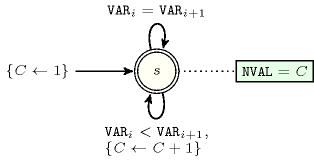## 5.187. increasing_nvalue

Origin
Constraint

$\mathrm{𝚒𝚗𝚌𝚛𝚎𝚊𝚜𝚒𝚗𝚐}_\mathrm{𝚗𝚟𝚊𝚕𝚞𝚎}\left(\mathrm{𝙽𝚅𝙰𝙻},\mathrm{𝚅𝙰𝚁𝙸𝙰𝙱𝙻𝙴𝚂}\right)$

Arguments
 $\mathrm{𝙽𝚅𝙰𝙻}$ $\mathrm{𝚍𝚟𝚊𝚛}$ $\mathrm{𝚅𝙰𝚁𝙸𝙰𝙱𝙻𝙴𝚂}$ $\mathrm{𝚌𝚘𝚕𝚕𝚎𝚌𝚝𝚒𝚘𝚗}\left(\mathrm{𝚟𝚊𝚛}-\mathrm{𝚍𝚟𝚊𝚛}\right)$
Restrictions
 $\mathrm{𝙽𝚅𝙰𝙻}\ge \mathrm{𝚖𝚒𝚗}\left(1,|\mathrm{𝚅𝙰𝚁𝙸𝙰𝙱𝙻𝙴𝚂}|\right)$ $\mathrm{𝙽𝚅𝙰𝙻}\le |\mathrm{𝚅𝙰𝚁𝙸𝙰𝙱𝙻𝙴𝚂}|$ $\mathrm{𝚛𝚎𝚚𝚞𝚒𝚛𝚎𝚍}$$\left(\mathrm{𝚅𝙰𝚁𝙸𝙰𝙱𝙻𝙴𝚂},\mathrm{𝚟𝚊𝚛}\right)$ $\mathrm{𝚒𝚗𝚌𝚛𝚎𝚊𝚜𝚒𝚗𝚐}$$\left(\mathrm{𝚅𝙰𝚁𝙸𝙰𝙱𝙻𝙴𝚂}\right)$
Purpose

The variables of the collection $\mathrm{𝚅𝙰𝚁𝙸𝙰𝙱𝙻𝙴𝚂}$ are increasing. In addition, $\mathrm{𝙽𝚅𝙰𝙻}$ is the number of distinct values taken by the variables of the collection $\mathrm{𝚅𝙰𝚁𝙸𝙰𝙱𝙻𝙴𝚂}$.

Example
 $\left(2,〈6,6,8,8,8〉\right)$ $\left(1,〈6,6,6,6,6〉\right)$ $\left(5,〈0,2,3,6,7〉\right)$

The first $\mathrm{𝚒𝚗𝚌𝚛𝚎𝚊𝚜𝚒𝚗𝚐}_\mathrm{𝚗𝚟𝚊𝚕𝚞𝚎}$ constraint (see Figure 5.187.1 for a graphical representation) holds since:

• The values of the collection $〈6,6,8,8,8〉$ are sorted in increasing order.

• $\mathrm{𝙽𝚅𝙰𝙻}=2$ is set to the number of distinct values occurring within the collection $〈6,6,8,8,8〉$.

##### Figure 5.187.1. Illustration of the first example of the Example slot: five variables ${V}_{1}$, ${V}_{2}$, ${V}_{3}$, ${V}_{4}$, ${V}_{5}$ respectively fixed to values 6, 6, 8, 8 and 8, and the corresponding number of distinct values $\mathrm{𝙽𝚅𝙰𝙻}=2$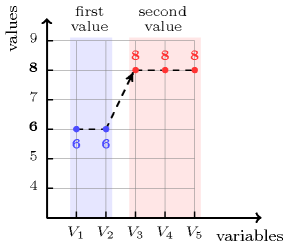Typical
 $|\mathrm{𝚅𝙰𝚁𝙸𝙰𝙱𝙻𝙴𝚂}|>1$ $\mathrm{𝚛𝚊𝚗𝚐𝚎}$$\left(\mathrm{𝚅𝙰𝚁𝙸𝙰𝙱𝙻𝙴𝚂}.\mathrm{𝚟𝚊𝚛}\right)>1$
Symmetry

One and the same constant can be added to the $\mathrm{𝚟𝚊𝚛}$ attribute of all items of $\mathrm{𝚅𝙰𝚁𝙸𝙰𝙱𝙻𝙴𝚂}$.

Arg. properties

Functional dependency: $\mathrm{𝙽𝚅𝙰𝙻}$ determined by $\mathrm{𝚅𝙰𝚁𝙸𝙰𝙱𝙻𝙴𝚂}$.

Algorithm

A complete filtering algorithm in a linear time complexity over the sum of the domain sizes is described in [BeldiceanuHermenierLorcaPetit10].

Reformulation

The $\mathrm{𝚒𝚗𝚌𝚛𝚎𝚊𝚜𝚒𝚗𝚐}_\mathrm{𝚗𝚟𝚊𝚕𝚞𝚎}$ constraint can be expressed in term of a conjunction of a $\mathrm{𝚗𝚟𝚊𝚕𝚞𝚎}$ and an $\mathrm{𝚒𝚗𝚌𝚛𝚎𝚊𝚜𝚒𝚗𝚐}$ constraints (i.e., a chain of non strict inequality constraints on adjacent variables of the collection $\mathrm{𝚅𝙰𝚁𝙸𝙰𝙱𝙻𝙴𝚂}$). But as shown by the following example, ${V}_{1}\in \left[1,2\right]$, ${V}_{2}\in \left[1,2\right]$, ${V}_{1}\le {V}_{2}$, $\mathrm{𝚗𝚟𝚊𝚕𝚞𝚎}$($2,〈{V}_{1},{V}_{2}〉$), this hinders propagation (i.e., the unique solution ${V}_{1}=1$, ${V}_{2}=2$ is not directly obtained after stating all the previous constraints).

A better reformulation achieving arc-consistency uses the $\mathrm{𝚜𝚎𝚚}_\mathrm{𝚋𝚒𝚗}$ constraint [PetitBeldiceanuLorca11] that we now introduce. Given $𝙽$ a domain variable, $𝚇$ a sequence of domain variables, and $𝙲$ and $𝙱$ two binary constraints, $\mathrm{𝚜𝚎𝚚}_\mathrm{𝚋𝚒𝚗}$$\left(𝙽,𝚇,𝙲,𝙱\right)$ holds if (1) $𝙽$ is equal to the number of $𝙲$-stretches in the sequence $𝚇$, and (2) $𝙱$ holds on any pair of consecutive variables in $𝚇$. A $𝙲$-stretch is a generalisation of the notion of stretch introduced by G. Pesant [Pesant01], where the equality constraint is made explicit by replacing it by a binary constraint $𝙲$, i.e., a $𝙲$-stretch is a maximal length subsequence of $𝚇$ for which the binary constraint $𝙲$ is satisfied on consecutive variables. $\mathrm{𝚒𝚗𝚌𝚛𝚎𝚊𝚜𝚒𝚗𝚐}_\mathrm{𝚗𝚟𝚊𝚕𝚞𝚎}$$\left(\mathrm{𝙽𝚅𝙰𝙻},\mathrm{𝚅𝙰𝚁𝙸𝙰𝙱𝙻𝙴𝚂}\right)$ can be reformulated as $\mathrm{𝚜𝚎𝚚}_\mathrm{𝚋𝚒𝚗}$$\left(\mathrm{𝙽𝚅𝙰𝙻},\mathrm{𝚅𝙰𝚁𝙸𝙰𝙱𝙻𝙴𝚂},=,\le \right)$.

Counting
 Length ($n$) 2 3 4 5 6 7 8 Solutions 6 20 70 252 924 3432 12870

Number of solutions for $\mathrm{𝚒𝚗𝚌𝚛𝚎𝚊𝚜𝚒𝚗𝚐}_\mathrm{𝚗𝚟𝚊𝚕𝚞𝚎}$: domains $0..n$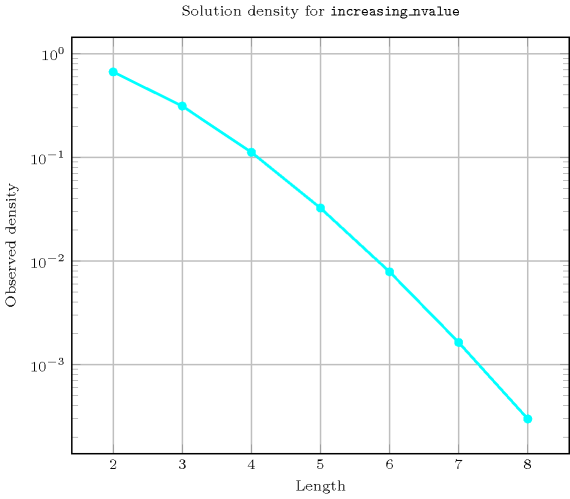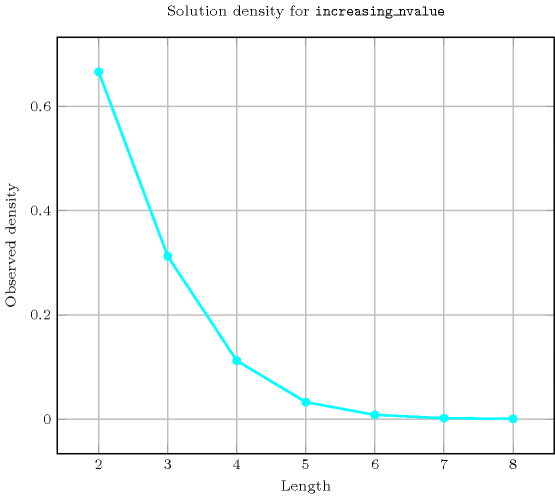Length ($n$)2345678
Total62070252924343212870
 Parameter value

13456789
23123060105168252
3-4301203508401764
4--56035014004410
5---61058404410
6----71681764
7-----8252
8------9

Solution count for $\mathrm{𝚒𝚗𝚌𝚛𝚎𝚊𝚜𝚒𝚗𝚐}_\mathrm{𝚗𝚟𝚊𝚕𝚞𝚎}$: domains $0..n$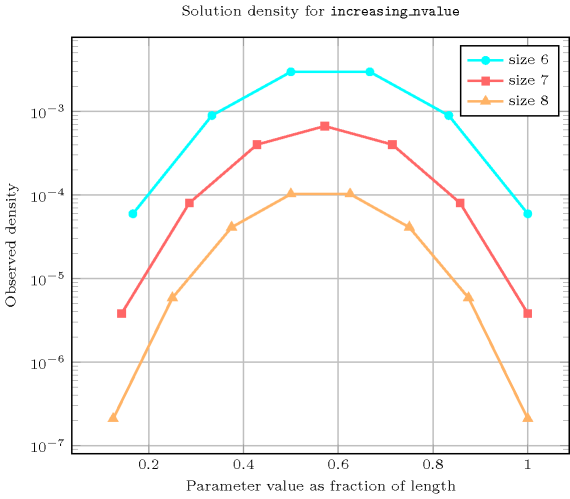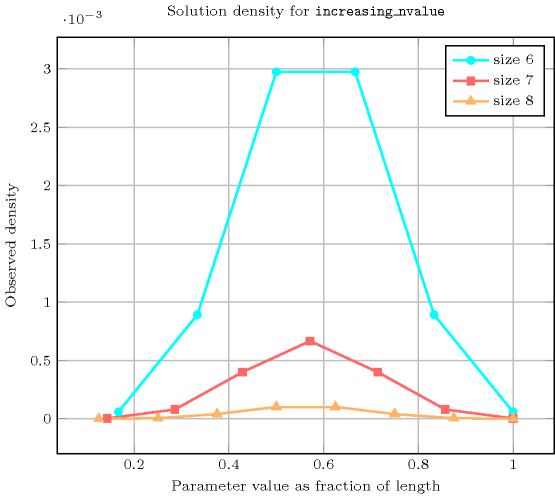Systems

implies: $\mathrm{𝚒𝚗𝚌𝚛𝚎𝚊𝚜𝚒𝚗𝚐}$ (remove $\mathrm{𝙽𝚅𝙰𝙻}$ parameter from $\mathrm{𝚒𝚗𝚌𝚛𝚎𝚊𝚜𝚒𝚗𝚐}_\mathrm{𝚗𝚟𝚊𝚕𝚞𝚎}$), $\mathrm{𝚗𝚟𝚊𝚕𝚞𝚎}$, $\mathrm{𝚗𝚟𝚒𝚜𝚒𝚋𝚕𝚎}_\mathrm{𝚏𝚛𝚘𝚖}_\mathrm{𝚜𝚝𝚊𝚛𝚝}$.

shift of concept: $\mathrm{𝚘𝚛𝚍𝚎𝚛𝚎𝚍}_\mathrm{𝚗𝚟𝚎𝚌𝚝𝚘𝚛}$ ($\mathrm{𝚟𝚊𝚛𝚒𝚊𝚋𝚕𝚎}$ replaced by $\mathrm{𝚟𝚎𝚌𝚝𝚘𝚛}$ and $\le$ replaced by $\mathrm{𝚕𝚎𝚡}_\mathrm{𝚕𝚎𝚜𝚜𝚎𝚚}$).

Keywords
Arc input(s)

$\mathrm{𝚅𝙰𝚁𝙸𝙰𝙱𝙻𝙴𝚂}$

Arc generator
$\mathrm{𝐶𝐿𝐼𝑄𝑈𝐸}$$↦\mathrm{𝚌𝚘𝚕𝚕𝚎𝚌𝚝𝚒𝚘𝚗}\left(\mathrm{𝚟𝚊𝚛𝚒𝚊𝚋𝚕𝚎𝚜}\mathtt{1},\mathrm{𝚟𝚊𝚛𝚒𝚊𝚋𝚕𝚎𝚜}\mathtt{2}\right)$

Arc arity
Arc constraint(s)
$\mathrm{𝚟𝚊𝚛𝚒𝚊𝚋𝚕𝚎𝚜}\mathtt{1}.\mathrm{𝚟𝚊𝚛}=\mathrm{𝚟𝚊𝚛𝚒𝚊𝚋𝚕𝚎𝚜}\mathtt{2}.\mathrm{𝚟𝚊𝚛}$
Graph property(ies)
$\mathrm{𝐍𝐒𝐂𝐂}$$=\mathrm{𝙽𝚅𝙰𝙻}$

Graph class
$\mathrm{𝙴𝚀𝚄𝙸𝚅𝙰𝙻𝙴𝙽𝙲𝙴}$

Graph model

Parts (A) and (B) of Figure 5.187.2 respectively show the initial and final graph associated with the first example of the Example slot. Since we use the $\mathrm{𝐍𝐒𝐂𝐂}$ graph property we show the different strongly connected components of the final graph. Each strongly connected component corresponds to a value that is assigned to some variables of the $\mathrm{𝚅𝙰𝚁𝙸𝙰𝙱𝙻𝙴𝚂}$ collection. The 2 following values 6 and 8 are used by the variables of the $\mathrm{𝚅𝙰𝚁𝙸𝙰𝙱𝙻𝙴𝚂}$ collection.

##### Figure 5.187.2. Initial and final graph of the $\mathrm{𝚒𝚗𝚌𝚛𝚎𝚊𝚜𝚒𝚗𝚐}_\mathrm{𝚗𝚟𝚊𝚕𝚞𝚎}$ constraint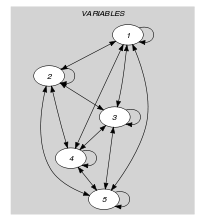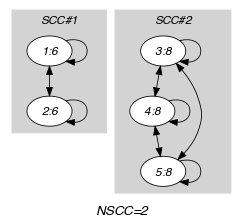(a) (b)
Automaton

A first systematic approach for creating an automaton that only recognises the solutions to the $\mathrm{𝚒𝚗𝚌𝚛𝚎𝚊𝚜𝚒𝚗𝚐}_\mathrm{𝚗𝚟𝚊𝚕𝚞𝚎}$ constraint could be to:

However this approach is not going to scale well in practice since the automaton associated with the $\mathrm{𝚗𝚟𝚊𝚕𝚞𝚎}$ constraint has a too big size. Therefore we propose an approach where we directly construct in a single step the automaton that only recognises the solutions to the $\mathrm{𝚒𝚗𝚌𝚛𝚎𝚊𝚜𝚒𝚗𝚐}_\mathrm{𝚗𝚟𝚊𝚕𝚞𝚎}$ constraint. Note that we do not have any formal proof that the resulting automaton is always minimum.

Without loss of generality, assume that the collection of variables $\mathrm{𝚅𝙰𝚁𝙸𝙰𝙱𝙻𝙴𝚂}$ contains at least one variable (i.e., $|\mathrm{𝚅𝙰𝚁𝙸𝙰𝙱𝙻𝙴𝚂}|\ge 1$). Let $l$, $m$, $n$, $\mathrm{𝑚𝑖𝑛}$ and $\mathrm{𝑚𝑎𝑥}$ respectively denote the minimum and maximum possible value of variable $\mathrm{𝙽𝚅𝙰𝙻}$, the number of variables of the collection $\mathrm{𝚅𝙰𝚁𝙸𝙰𝙱𝙻𝙴𝚂}$, the smallest value that can be assigned to the variables of $\mathrm{𝚅𝙰𝚁𝙸𝙰𝙱𝙻𝙴𝚂}$, and the largest value that can be assigned to the variables of $\mathrm{𝚅𝙰𝚁𝙸𝙰𝙱𝙻𝙴𝚂}$. Let $s=\mathrm{𝑚𝑎𝑥}-\mathrm{𝑚𝑖𝑛}+1$ denote the total number of potential values. Clearly, the maximum number of distinct values that can be assigned to the variables of the collection $\mathrm{𝚅𝙰𝚁𝙸𝙰𝙱𝙻𝙴𝚂}$ cannot exceed the quantity $d=min\left(m,n,s\right)$. The $\frac{s·\left(s+1\right)}{2}-\frac{\left(s-d\right)·\left(s-d+1\right)}{2}+1$ states of the automaton that only accepts solutions to the $\mathrm{𝚒𝚗𝚌𝚛𝚎𝚊𝚜𝚒𝚗𝚐}_\mathrm{𝚗𝚟𝚊𝚕𝚞𝚎}$ constraint can be defined in the following way:

• We have an initial state labelled by ${s}_{00}$.

• We have $\frac{s·\left(s+1\right)}{2}-\frac{\left(s-d\right)·\left(s-d+1\right)}{2}$ states labelled by ${s}_{ij}$ $\left(1\le i\le d,i\le j\le s\right)$. The first index $i$ of a state ${s}_{ij}$ corresponds to the number of distinct values already encountered, while the second index $j$ denotes the the current value (i.e., more precisely the index of the current value, where the minimum value has index 1).

Terminal states depend on the possible values of variable $\mathrm{𝙽𝚅𝙰𝙻}$ and correspond to those states ${s}_{ij}$ such that $i$ is a possible value for variable $\mathrm{𝙽𝚅𝙰𝙻}$. Note that we assume no further restriction on the domain of $\mathrm{𝙽𝚅𝙰𝙻}$ (otherwise the set of accepting states needs to be reduced in order to reflect the current set of possible values of $\mathrm{𝙽𝚅𝙰𝙻}$). Three classes of transitions are respectively defined in the following way:

1. There is a transition, labelled by $\mathrm{𝑚𝑖𝑛}+j-1$, from the initial state ${s}_{00}$ to the state ${s}_{1j}$ $\left(1\le j\le s\right)$.

2. There is a loop, labelled by $\mathrm{𝑚𝑖𝑛}+j-1$ for every state ${s}_{ij}$ $\left(1\le i\le d,i\le j\le s\right)$.

3. $\forall i\in \left[1,d-1\right],\forall j\in \left[i,s\right],\forall k\in \left[j+1,s\right]$ there is a transition labelled by $\mathrm{𝑚𝑖𝑛}+k-1$ from ${s}_{ij}$ to ${s}_{i+1k}$.

We respectively have $s$ transitions of class 1, $\frac{s·\left(s+1\right)}{2}-\frac{\left(s-d\right)·\left(s-d+1\right)}{2}$ transitions of class 2, and $\frac{\left(s-1\right)·s·\left(s+1\right)}{6}-\frac{\left(s-d\right)·\left(s-d+1\right)·\left(s-d+2\right)}{6}$ transitions of class 3.

Note that all states ${s}_{ij}$ such that $i+s-j can be discarded since they do not allow to reach the minimum number of distinct values required $l$.

Part (A) of Figure 5.187.3 depicts the automaton associated with the $\mathrm{𝚒𝚗𝚌𝚛𝚎𝚊𝚜𝚒𝚗𝚐}_\mathrm{𝚗𝚟𝚊𝚕𝚞𝚎}$ constraint of the first example of the Example slot. For this purpose, we assume that variable $\mathrm{𝙽𝚅𝙰𝙻}$ is fixed to value 2 and that variables of the collection $\mathrm{𝚅𝙰𝚁𝙸𝙰𝙱𝙻𝙴𝚂}$ take their values within interval $\left[6,8\right]$. Part (B) of Figure 5.187.3 represents the simplified automaton where all states that do not allow to reach an accepting state were removed. The corresponding $\mathrm{𝚒𝚗𝚌𝚛𝚎𝚊𝚜𝚒𝚗𝚐}_\mathrm{𝚐𝚕𝚘𝚋𝚊𝚕}_\mathrm{𝚌𝚊𝚛𝚍𝚒𝚗𝚊𝚕𝚒𝚝𝚢}$ constraint holds since the corresponding sequence of visited states, ${s}_{00}$ ${s}_{11}$ ${s}_{11}$ ${s}_{23}$ ${s}_{23}$ ${s}_{23}$, ends up in an accepting state (i.e., accepting states are denoted graphically by a double circle).

##### Figure 5.187.3. Automaton – Part (A) – and simplified automaton – Part (B) – of the $\mathrm{𝚒𝚗𝚌𝚛𝚎𝚊𝚜𝚒𝚗𝚐}_\mathrm{𝚗𝚟𝚊𝚕𝚞𝚎}$$\left(2,〈6,6,8,8,8〉\right)$ constraint of the first example of the Example slot: the path corresponding to the second argument $〈\mathbf{6},\mathbf{6},\mathbf{8},\mathbf{8},\mathbf{8}〉$ is depicted by thick orange arcs, where the self-loop on state ${s}_{23}$ is applied twice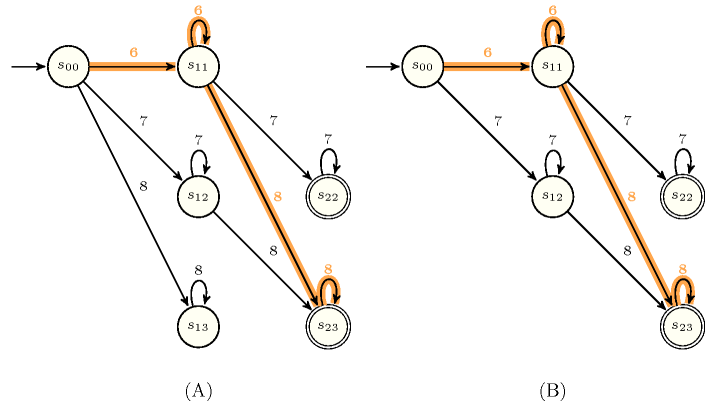Figure 5.187.4 depicts a second deterministic automaton with one counter associated with the $\mathrm{𝚒𝚗𝚌𝚛𝚎𝚊𝚜𝚒𝚗𝚐}_\mathrm{𝚗𝚟𝚊𝚕𝚞𝚎}$ constraint, where the argument $\mathrm{𝙽𝚅𝙰𝙻}$ is unified to the final value of the counter.

##### Figure 5.187.4. Automaton (with one counter) of the $\mathrm{𝚒𝚗𝚌𝚛𝚎𝚊𝚜𝚒𝚗𝚐}_\mathrm{𝚟𝚊𝚕𝚞𝚎}$ constraint for a non-empty collection of variables